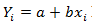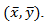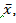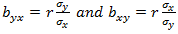Goseeko blog# What is regression analysis?

## Overview(regression analysis)

First time the term regression analysis was used by a British Biometrician Sir Francis Galton. He found that the offspring of tall or short parents tend to regress to the average height.

The term ‘regression’ means some sort of functional relationship between two or more related variables.

Regression is the estimation of unknown values of one variable from known values of another variable. We can also state the regression analysis as below-

Regression is the measure of average relationship between independent and dependent variable. We can used for two or more than two variables. There are two types of variables in this analysis-

1. Independent variable

2. Dependent variable

The variable which is used for prediction is called independent variable.

It is known as predictor or regressor.

The variable whose value is predicted by independent variable is called dependent variable or regressed or explained variable.

The scatter diagram shows relationship between independent and dependent variable, then the scatter diagram will be more or less concentrated round a curve, which is called the curve of regression.

When we find the curve as a straight line then it is known as line of regression and the regression is called linear regression.

## What is correlation?

When two variables are related in such a way that change in the value of one variable affects the value of the other variable, then these two variables are said to be correlated and there is correlation between two variables.

Example- Height and weight of the persons of a group.

The correlation is said to be perfect correlation if two variables vary in such a way that their ratio is constant always.

## Curve and regression of equation

If the scatter diagram indicates some relationship between two variables and , then the dots of the scatter diagram will be concentrated round a curve. This curve is called the curve of regression.

Regression analysis is the method used for estimating the unknown values of one variable corresponding to the known value of another variable.

The mathematical equation of the regression curve is called regression equation.

Note- regression line is the best fit line which expresses the average relation between variables.

When we get the curve as a straight line that curve is called a line of regression. A line of regression is the straight line which gives the best fit in the least square sense to the given frequency.

## Difference between regression and correlation

1. Correlation is the linear relationship between two variables and regression is the average relationship between two or more variables.

2. There are only limited applications of correlation as it gives the strength of linear relationship and the regression is to predict the value of the dependent varibale for the given values of independent variables.

3. Correlation does not consider dependent and independent variables and regression consider one dependent variable and other indpendent variables.

## Equation of the line of regression

Let

is the equation of the line of y on x.

Letbe the estimated value of yi for the given value of x = xi

According to the principle of least squares, we have the determined ‘a’ and ‘b’, sum of squares of deviations of observed values of y from expected values of y,

That means-

Or

Is minimum.

Form the concept of maxima and minima, we partially differentiate U with respect to ‘a’ and ‘b’ and equate to zero.

Which means

And

These equations (3) and (4) are known as normal equation for straight line.

Divide equation (3) by n, we get-

This indicates that the regression line of y on x passes through the point.

As we know that-

The variance of variable x can be expressed as-

Now, dividing equation (4) by n, we get-

from the equations (6), (7) and (8)

Multiply (5) by, we get-

Subtracting equation (10) from equation (9), we get-

Since ‘b’ is the slope of the line of regression y on x and the line of regression passes through the point, so that the equation of the line of regression of y on x is-

Which is known as regression line of y on x.

Note-

1.are the coefficients of regression.

## Solved examples of regression analysis

Example: Find the regression line of y on x for the given dataset.

Sol.

Let y = a + bx is the line of regression of y on x, where ‘a’ and ‘b’ are given as-

Construct the following table

Using the above equations we get-

So that on solving these both equations-

a = 15.49 and b = -0.675

Hence, the regression line will be

y = 15.49 – 0.675x

Interested in learning about similar topics? Here are a few hand-picked blogs for you!